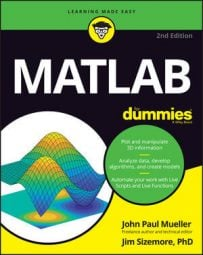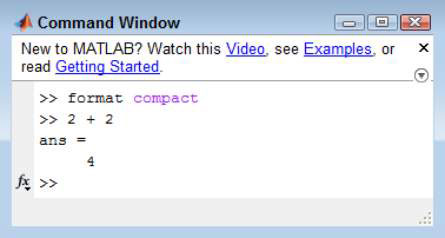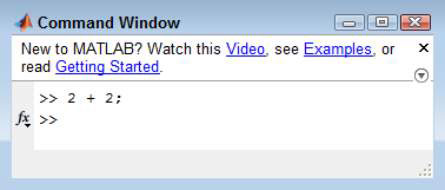##### MATLAB For DummiesMATLAB can perform some pretty tricky calculations. While that is all well and good, don’t neglect the ability of MATLAB to act as a calculator. If you want to take advantage of this, you will need to know how to use the Command window.

## Changing the Command window formatting

For example, if you don’t want the extra space between lines that MATLAB provides by default, you can type format compact and press Enter to get rid of it.

In fact, try typing that command now. When you type format compact and press Enter, you don’t see any output. However, the next formula you type shows the difference. Type 2 + 2 and press Enter. You see that the extra spaces between lines are gone.MATLAB provides a number of format commands. Each of them begins with the keyword format, followed by an additional instruction. Here is a list of the instructions you can type:

• short: All floating-point output has at least one whole number, a decimal point, and four decimal values, such as 4.2000.

• long: All floating-point output has at least one whole number, a decimal point, and 15 decimal values, such as 4.200000000000000.

• shorte: All floating-point output uses exponential format with four decimal places, such as 4.2000e+00.

• longe: All floating-point output uses exponential format with 15 decimal places, such as 4.200000000000000e+00.

• shortg: All output uses a short general format, such as 4.2, with five digits of space.

• long: All output uses a long general format, such as 4.2, with 15 digits of space.

• shorteng: All floating-point output uses exponential format with four decimal places and powers in groups of three, such as 4.2000e+000.

• longeng: All floating-point output uses exponential format with 14 decimal places and powers in groups of three, such as 4.20000000000000e+000.

• hex: All output is in hexadecimal format, such as 4010cccccccccccd.

• +: All output is evaluated for positive or negative values, so that the result contains just a + or - sign, such as + when using the formula 2 * 2.1.

• bank: All output provides two decimal places, even for integer calculations, such as 4.20.

• rat: All output is presented as a ratio of small integers, such as 21/5 for 4.2.

• compact: All output appears in single-spaced format.

• loose: All output appears in double-spaced format.

## Suppressing Command window output

When performing most experiments, you want to see the result of your actions. However, sometimes you really don’t want to keep seeing the results in the Command window when you can just as easily look in the Workspace window for the result. In these cases, you can follow a command with a semicolon (;) and the Command window output is suppressed.

For example, try typing 2 + 2; and pressing Enter (note the semicolon at the end of the command).Now look at the Workspace window. The results are shown there just as you would expect. This technique is often used when you have a complex set of formulas to type and you don’t want to see the intermediate results or when working with large matrices.

Of course, you also want to use this approach when you create scripts so that the script user isn’t bombarded by the results that will appear as the script runs. Anytime you stop using the semicolon at the end of the command, you start seeing the results again.